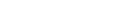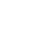# Aggregation functions

## Statistical functions

Function NameDescription
avg()Returns an average value across the group.
countif()Returns a count of rows for which Predicate evaluates to true
count()Returns a count of the group without/with a predicate.
dcount()Returns an estimate for the number of distinct values that are taken by a scalar expression in the summary group.
dcountif()Returns an estimate of the number of distinct values of Expr of rows for which Predicate evaluates to true.
max()Returns the maximum value across the group.
min()Returns the minimum value across the group.
sum()Calculates the sum of Expr across the group.
histogram()Returns a timeseries heatmap chart across the group.
topk()calculates the top values of Expr across the group in a dataset
percentile()calculates the requested percentiles of the group and produces a timeseries chart.
variance()Calculates the variance of Expr across the group.
stdev()Calculates the standard deviation of Expr across the group.

## avg()

Calculates the average (arithmetic mean) of Expr across the group.

### Arguments

• Expr: Expression that will be used for aggregation calculation.

### Returns

The average value of Expr across the group.

### Example

`avg (Expr)`

## countif()

Returns a count of rows for which Predicate evaluates to `true`. Can only be used only in context of aggregation inside summarize.

### Arguments

Predicate: Expression that will be used for aggregation calculation. Predicate can be any scalar expression with return type of bool (evaluating to true/false).

### Returns

Returns a count of rows for which Predicate evaluates to `true.`

### Example

`countif (Predicate)`

## count()

Returns a count of the records per summarization group (or in total, if summarization is done without grouping).

### Returns

Returns a count of the records per summarization group.

### Example

`count ( )`

## dcount()

Returns an estimate for the number of distinct values that are taken by a scalar expression in the summary group.

### Arguments

• Expr: A scalar expression whose distinct values are to be counted.

### Returns

Returns an estimate of the number of distinct values of `Expr` in the group.

### Example

`dcount (Expr)`

## dcountif()

Returns an estimate of the number of distinct values of Expr of rows for which Predicate evaluates to `true.`

• Can be used only in context of aggregation inside summarize.

### Arguments

• Expr: Expression that will be used for aggregation calculation.

• Predicate: Expression that will be used to filter rows.

### Returns

Returns an estimate of the number of distinct values of Expr of rows for which Predicate evaluates to `true` in the group.

### Example

`dcountif (Expr, Predicate)`

## max()

Returns the maximum value across the group.

• Can be used only in context of aggregation inside summarize

### Arguments

• Expr: Expression that will be used for aggregation calculation.

### Returns

The maximum value of Expr across the group.

### Example

`max (Expr)`

## min()

Returns the minimum value across the group.

### Arguments

• Expr: Expression that will be used for aggregation calculation.

### Returns

The minimum value of Expr across the group.

### Example

`min (Expr)`

## sum()

Calculates the sum of Expr across the group.

• Can be used only in context of aggregation inside summarize

### Arguments

• Expr: Expression that will be used for aggregation calculation.

### Returns

The sum value of Expr across the group.

### Example

`sum (Expr)`

## histogram()

Returns a timeseries heatmap chart across the group

### Arguments

• Expr: Expression that will be used for aggregation calculation.

### Returns

Returns a timeseries heatmap chart across the group

### Example

`histogram(Expr)`

## topk()

calculates the top values of Expr across the group in a dataset

### Arguments

• Expr: Expression that will be used for aggregation calculation.

### Returns

• A seperate result for each group plotted on a timeseries chart.

### Example

`topk(Expr)`

## percentile(), percentiles_array()

Calculates the requested percentile of the group and produces a timeseries chart.

This function can be used only in context of aggregation inside summarize

### Arguments

• Expr: Expression that will be used for aggregation calculation.
• Percentile: A double constant that specifies the percentile.

### Returns

A seperate result for each group plotted on a horizonal bar chart, allowing for visual comparison across the groups.

### Examples

`percentile(Expr, percentile)`
`percentiles_array (Expr, Percentile1 [,Percentile2])`

## variance()

Calculates the variance of Expr across the group.

• Can be used only in context of aggregation inside summarize

### Arguments

• Expr: Expression that will be used for aggregation calculation.

### Returns

The variance value of Expr across the group.

### Eample

`variance (Expr)`

## stdev()

Calculates the standard deviation of Expr across the group.

• Can be used only in context of aggregation inside summarize

### Arguments

• Expr: Expression that will be used for aggregation calculation.

### Returns

The standard deviation value of Expr across the group.

### Example

`stdev (Expr)`Unable to retrieve cloud status